# Multiplication of Fractions

Multiplication of Fractions
Go back to  'Fractions'

 1 Introduction to Multiplying Fractions 2 Visually Representing the Multiplication of Fractions 3 Multiplying with Whole Numbers 4 Multiplying Two Equivalent Fractions 5 Multiplying Two Improper Fractions 6 Multiplying Two Mixed Fractions 7 Solved Examples on Multiply Fractions 8 Practice Questions on Multiplying Fractions 9 Tips and Tricks 10 Important Notes on Multiply Fractions 11 Maths Olympiad Sample Papers 12 Frequently Asked Questions (FAQs)

We at Cuemath believe that Math is a life skill. Our Math Experts focus on the “Why” behind the “What.” Students can explore from a huge range of interactive worksheets, visuals, simulations, practice tests, and more to understand a concept in depth.

and experience Cuemath’s LIVE Online Class with your child.

## Introduction to Multiplying Fractions

Fractions is an important topic that takes time and practice to master the concepts.

As a student, you may find it challenging initially.

We don't want you to worry as we, at Cuemath, understand how this topic can be confusing for you.

We are here to help you understand this concept in a clear way.

Let us now learn how to multiply fractions.

To multiply any two fractions, we have to follow the steps given below:

1. Multiply the numerators
2. Multiply the denominators
3. Reduce the resulting fraction to its lowest term

Let's see how this is done with an example.

Let's multiply the following fractions:

\begin{align} \frac{2}{3}\times\frac{4}{5} \end{align}

The steps to find the solution are:

1. Multiply the numerators 2 x 4 = 8
2. Multiply the denominators 3 x 5 = 15
3. Since, its already in it's lowest term we can leave it as is.

\begin{align} \frac{2}{3}\times \frac{4}{5} = \frac{2\times 4}{3\times 5}= \frac{8}{15} \end{align}

Wasn't this easy to solve?

Let's understand how to multiply fractions in detail now.

## Visually Representing the Multiplication of Fractions

At Cuemath, we are committed to making you understand the exact reasons and steps behind certain Math concepts.

Now, we will show you a visual representation for multiplication of fractions to help you understand this concept.

Visualizing multiplication of fractions using fractional squares is a very simple and easy method.

Do you know what are fractional squares?

Fractional squares are a representation of the given fraction in the form of squares where the numerator is indicated as a shaded portion.

Let us see how to multiply a fraction using fractional squares.

Let's multiply these two fractions:

\begin{align} \frac{2}{3}\times\frac{1}{2} \end{align}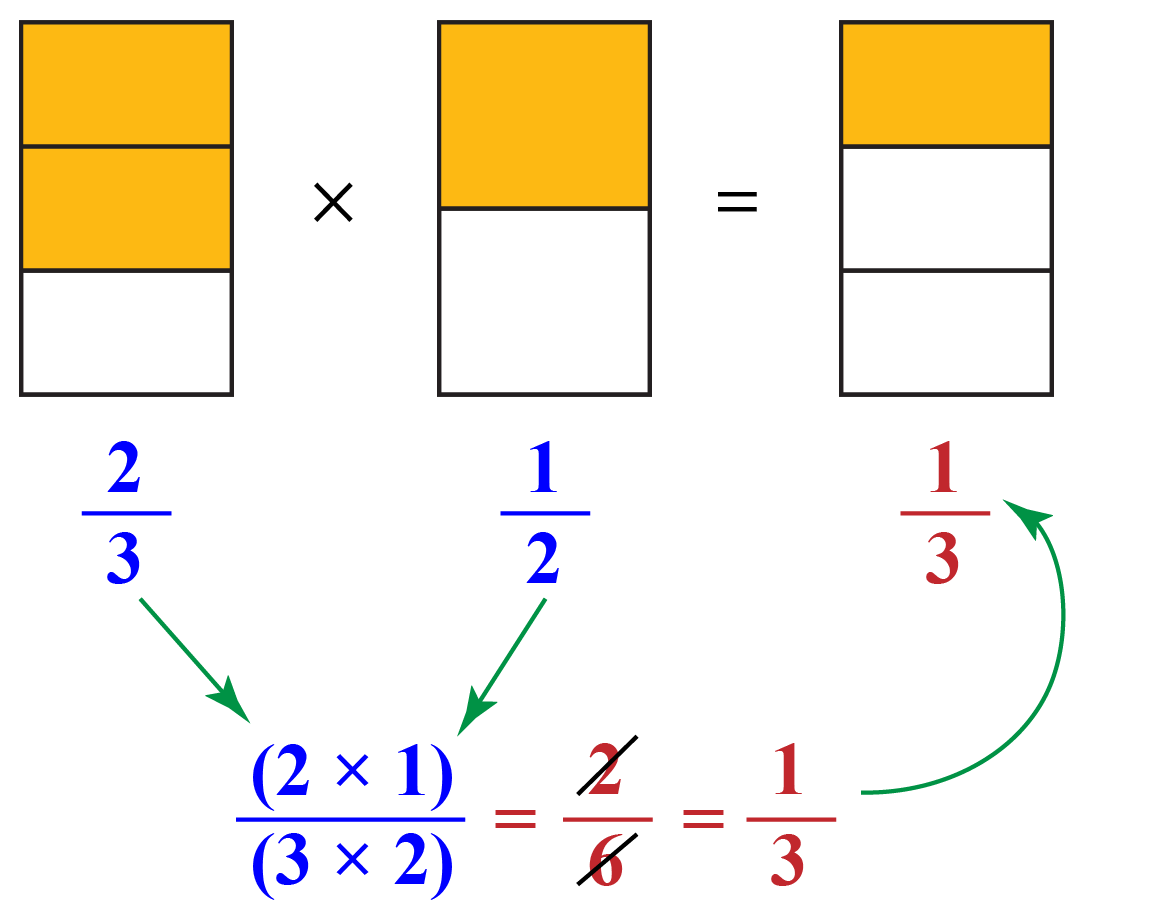You can read this out as:

Two parts of three $$\times$$ one part of two = 1 part of three

In the above figure, the fractional square on the left has 2 orange-shaded parts out of 3 equal sections.

This orange area represents two thirds of the fraction square.

Similarly, the second shaded area represents a half of the fraction square.

By multiplying these two fraction squares, we get  \begin{align}\frac{2}{6}\end{align}

This can be reduced to it's simplest form and represented by one part of three squares.

Thus,

\begin{align} \frac{2}{3}\times\frac{1}{2} = \frac{2}{6} =\frac{1}{3}\end{align}

Now that you have the insight into multiplying fractions, let us explore this topic further.

## Multiplying with Whole Numbers

Multiplying fractions by whole numbers is a easy to start concept.

Let us consider this example: $$4\times\frac{2}{3}$$ to understand the basic.

We will first represent this example using fractional squares.

Four times two-thirds is represented as: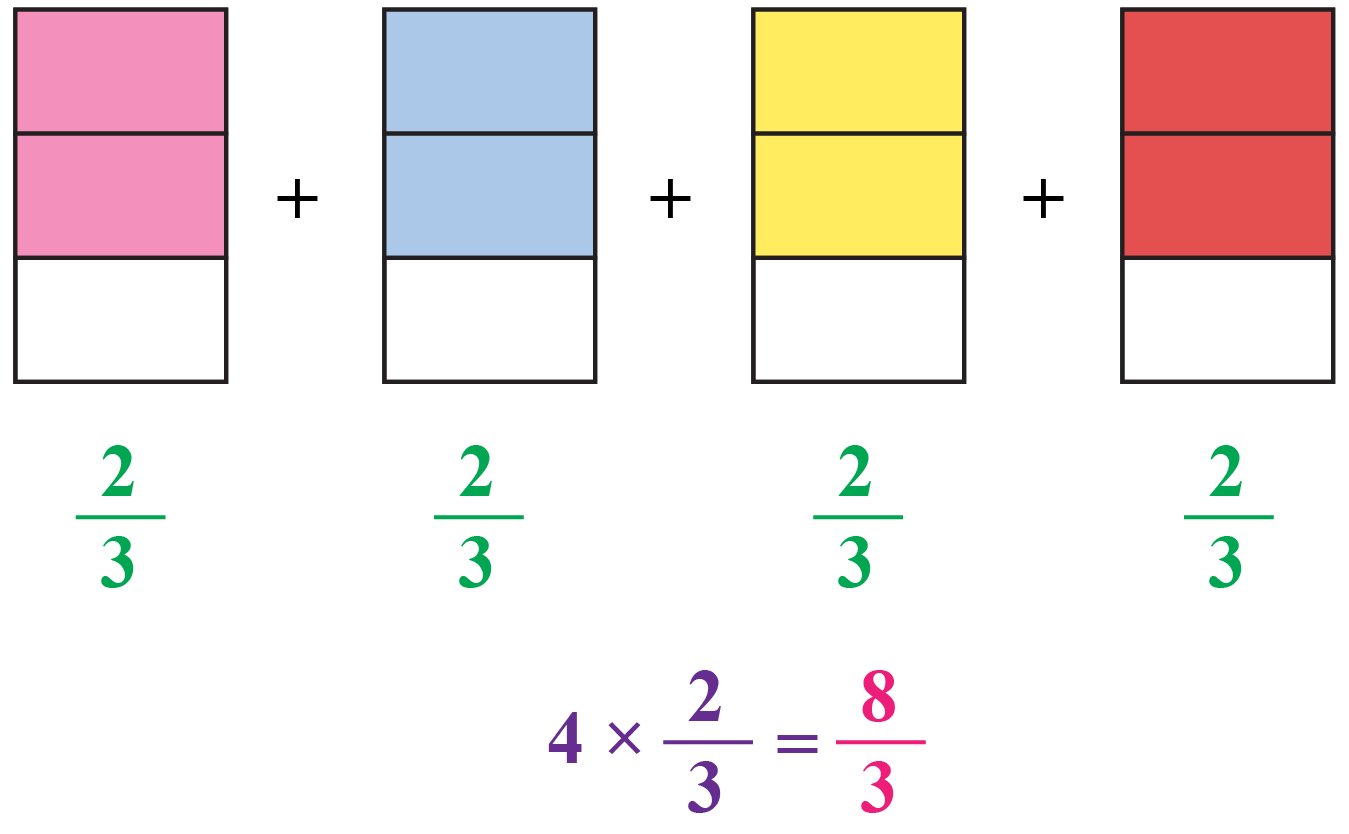Now let us change the improper fraction obtained into a mixed fraction.

\begin{align} \frac{8}{3}= 2\frac{2}{3}\end{align}

Finally, we get: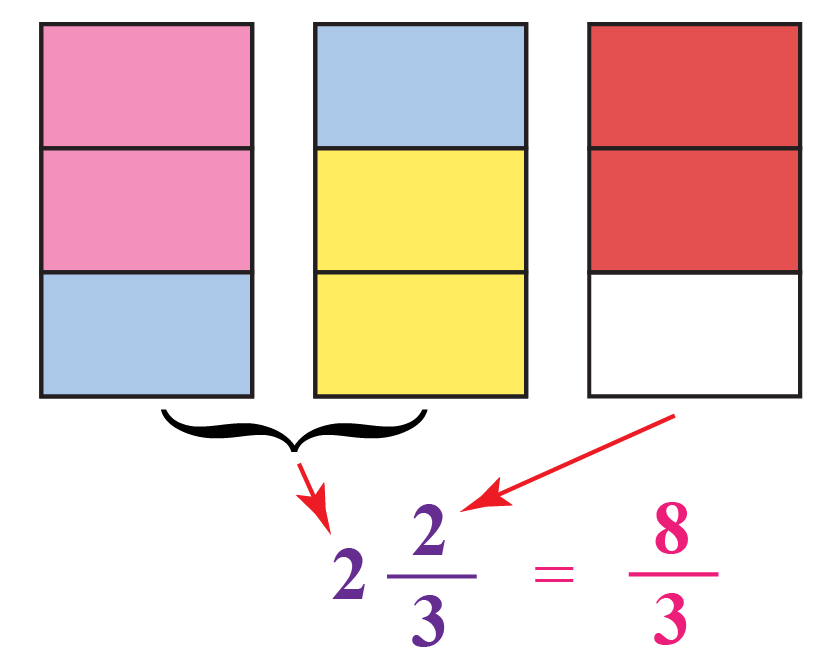Two whole and two thirds which is equal to \begin{align}\frac{8}{3}\end{align}

## Multiplying Two Equivalent Fractions

Multiplication of two equivalent fractions is always easy and intresting.

As an example, let us take \begin{align}\frac{2}{3}\times \frac{4}{6}\end{align}

Here, \begin{align}\frac{2}{3}\ and\ \frac{4}{6}\end{align} are equivalent fractions.

To multiply them, we will do the following:

• Multiply the numerators together: 2 x 4 = 8
• Multiply the denominators together: 3 x 6 = 18
• Then, reduce it to its lowest form.

\begin{align} \frac{2}{3}\times\frac{4}{6} = \frac{2\times 4}{3\times 6} = \frac{8}{18} \end{align}

You can observe that when you multiply two equivalent fractions, you finally also get the answer of equivalent fraction.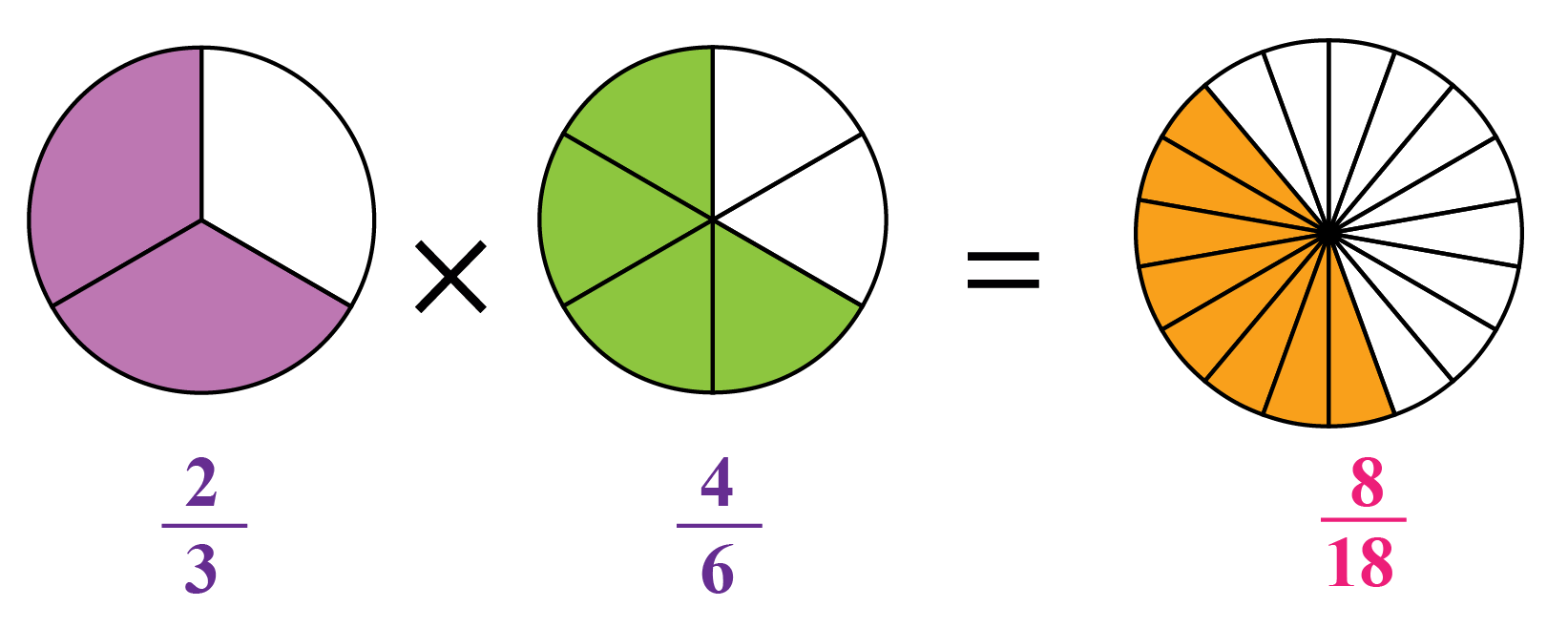\begin{align}\frac{2\times 4}{3\times 6} = \frac{8}{18}\end{align}

## Multiplying Two Improper Fractions

Now let's understand multiplication of improper fractions.

We already know that an improper fraction is one where the numerator is bigger than the denominator.

When multiplying two improper fractions, we frequently end up with an improper fraction.

We can understand this better by going through an example.

Multiply \begin{align}\frac{3}{2} \times \frac{7}{5} \end{align}

STEP 1: Multiply \begin{align}\frac{3}{2} \times \frac{7}{5} \end{align} which are two improper fractions.

\begin{align} \frac{3}{2} \times \frac{7}{5} \end{align}

STEP 2: Multiply the numerators and denominators.

\begin{align} \frac{3\times 7}{2\times 5} \end{align}

STEP 3: We get \begin{align} \frac{21}{10} \end{align} which cannot be reduced to its lowest terms. Hence, the answer is:

\begin{align} \frac{21}{10} \end{align}

Multiply Fractions Calculator:

Multipliy Fraction Calculator to show the Multiplication of Proper Fractions

## Multiplying Two Mixed Fractions

Mixed numbers are numbers that have a whole number and a fraction, like \begin{align}2\frac{1}{2}\end{align}

When multiplying mixed numbers, you have to change the mixed number into an improper fraction before you multiply.

For example, if the number is \begin{align}2\frac{1}{3}\end{align}, you should this to \begin{align}\frac{7}{3}\end{align} before you multiply.

You may also have to change the answer back to a mixed number after you are done multiplying.

Let's consider an example.

Multiply \begin{align}2\frac{1}{3}\end{align} and \begin{align}2\frac{1}{4}\end{align}

The steps we will follow to solve this example are:

Step 1: Change the given mixed fractions to improper fraction

Step 2: Multiply the numerators of the improper fracions

Step 3: Multiply the denomerators

Step 4: Convert the answer back to mixed fractions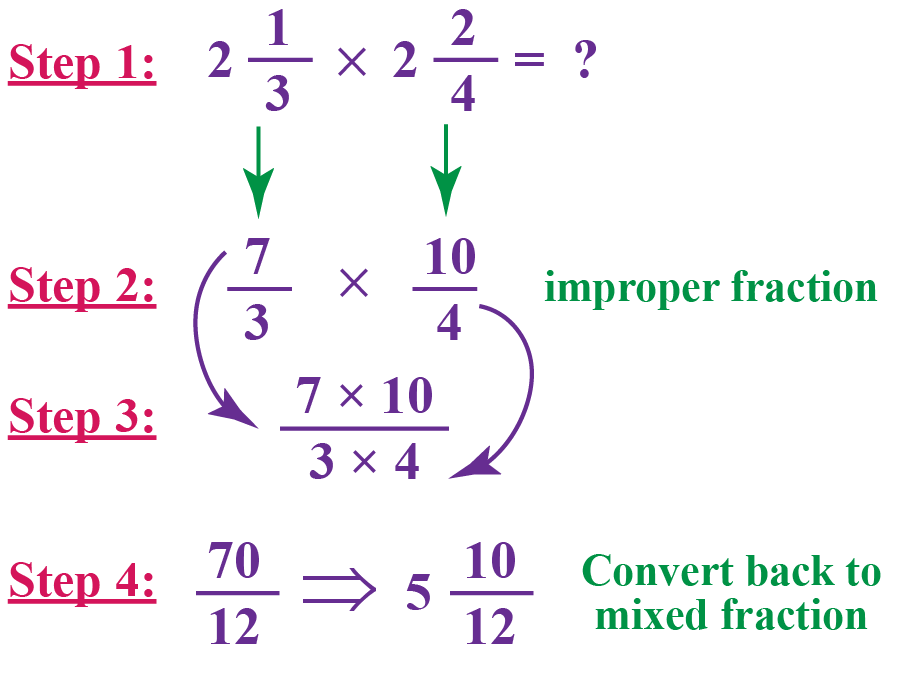Now did you understand how it is done?

Let us try some more multiply fraction examples,Important Notes
1. Generally, students simplify a fraction after multiplication.
However to make calculations easier, check if the two fractions to be multiplied are already in their lowest forms.
If not, first simplify them and then multiply.
This will make it easier to calculate and the answer will be the same.
E.g.\begin{align} \frac{4}{12}\times \frac{5}{13}\end{align} will be difficult to multiply directly.
But if you simplify the fraction first, we get: \begin{align}\frac{1}{3}\times \frac{5}{13} = \frac{5}{39}\end{align}
2. Simplification can also be done across two fractions.
If there is a common factor between the numerator of one of the fractions and the denominator of the other fraction, you can simplify them and proceed.
E.g.\begin{align}\frac{5}{28}\times \frac{7}{9}\end{align} can be simplified to \begin{align}\frac{5}{4}\times \frac{1}{9}\end{align} before multiplying.

Help your child score higher with Cuemath’s proprietary FREE Diagnostic Test. Get access to detailed reports, customised learning plans and a FREE counselling session. Attempt the test now.

## Solved Examples on Multiplying Fractions

 Example 1

Daniel was hungry and wanted to eat a pie.

There was only \begin{align} \frac{5}{8}^{th} \end{align} of the pie left in the fridge.

Daniel ate \begin{align}\frac{1}{4}\end{align} of the leftover pie.

How much of the pie did he have now?

Did he have less than \begin{align}\frac{1}{4}\end{align} of the pie or more than \begin{align}\frac{1}{2}\end{align} of the remaining pie?Solution:

The fraction of pie left in the fridge = \begin{align}\frac{5}{8}\end{align}

The amount Daniel ate from the left over pie = \begin{align}\frac{1}{4}\end{align}

Hence, the left over amount from the pie in the fridge will be  = \begin{align}\frac{1}{4}\ of\ \frac{5}{8}\end{align}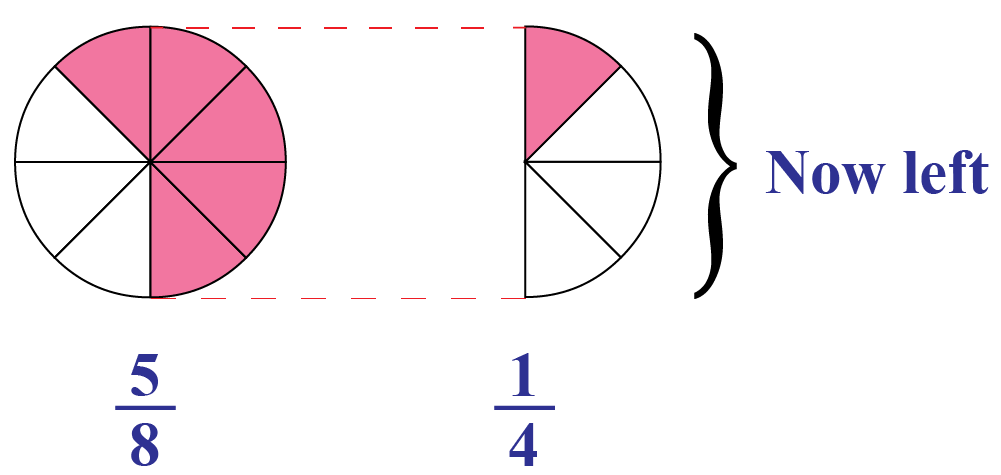\begin{align} \frac{5}{8} \times \frac{1}{4} = \frac{5}{32} \end{align}

This shows that, Daniel now has less than \begin{align}\frac{1}{4}\end{align} of the remaining pie.

 $$\therefore$$ Daniel ate \begin{align}\frac{5}{32}\end{align} of the Pie.
 Example 2

Jishita's brother is \begin{align}1\frac{1}{2}\end{align} meters tall.

Jishita is \begin{align}1\frac{1}{5}\end{align} times more taller than her brother.

How tall is Jishita?

Solution:

Jishita is \begin{align}1\frac{1}{5}\end{align} times \begin{align}1\frac{1}{2}\end{align} meters tall.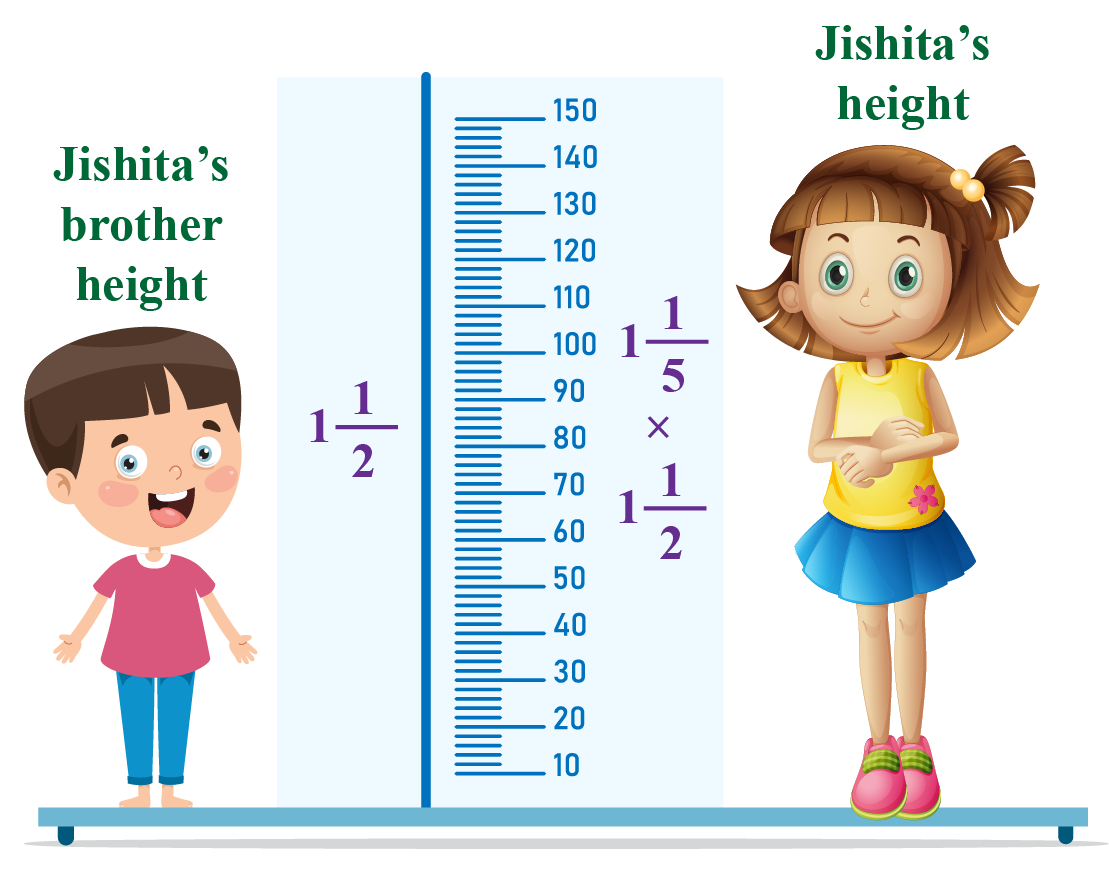Let assume Jishita's height is = $$x$$ meters

Height of Jishita's brother  = \begin{align}1\frac{1}{2}\end{align} meters

Thus, Jishita's height will be:

\begin{align} x = 1\frac{1}{2} \times 1\frac{1}{5} = \frac{3}{2} \times \frac{6}{5} \end{align}

\begin{align} = \frac{9}{5} \end{align}

\begin{align} = 1\frac{4}{5} \end{align}

 $$\therefore$$ Jishita is \begin{align} = 1\frac{4}{5} \end{align} meters tall.
 Example 3

Thomas is growing a beautiful garden.

\begin{align}\frac{1}{6}\end{align} of the garden is used for growing petunias.

\begin{align}\frac{3}{4}\end{align} of the petunias are pink.

What fraction of the garden has pink petunias?Solution:

The fraction of the garden growing petunias  = \begin{align}\frac{1}{6}\end{align}

Fraction of pink petunias  = \begin{align}\frac{3}{4}\end{align}

Fraction of the garden growing pink petunias  =  \begin{align}\frac{1}{6}\times \frac{3}{4} = \frac{1}{8}\end{align}

 $$\therefore$$ Thomas planted pink petunias in \begin{align}\frac{1}{8}\end{align} of the garden.
 Example 4

Last week, Lara spent 3 hours watching television.

Riely watched television for \begin{align}\frac{2}{3}\end{align} as many hours as Lara.

How many hours did Riely spend watching television?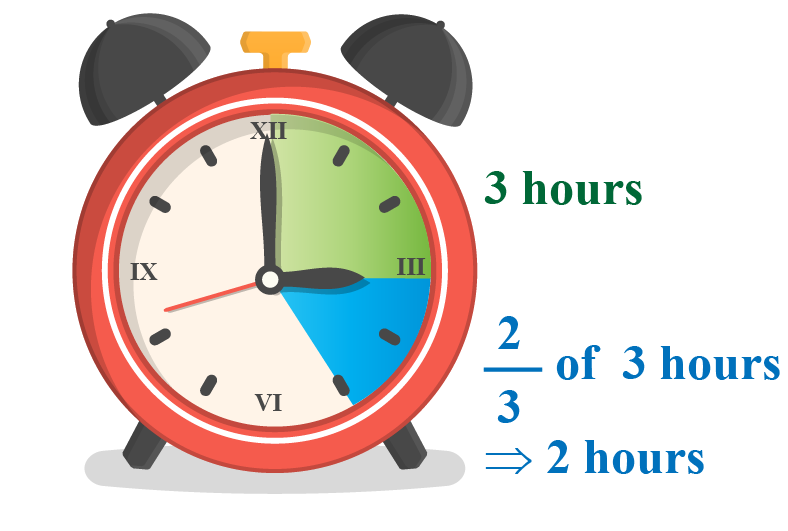Solution:

No of hours Lara watched TV = 3 hours

No of hours Riely watched TV is given by:

\begin{align}x=\frac{2}{3} \times 3 \end{align}

Let's write 3 as an improper fraction.

\begin{align}\frac{3}{1}\end{align}

Multiply the numerators and the denominators.

\begin{align} \frac{2}{3} \times \frac{3}{1} = \frac{2\times 3}{3\times 1} = \frac{6}{3} \end{align}

Simplify the product.

\begin{align} \frac{6}{3} = 2 \end{align}

 $$\therefore$$ Riely spent 2 hours watching TV.
 Example 5

Emma runs \begin{align}\frac{12}{5}\end{align} times faster than Dheeraj, who runs \begin{align}\frac{7}{3}\end{align} times faster than Hasmitha.

How fast does Emma run compared to Hasmitha?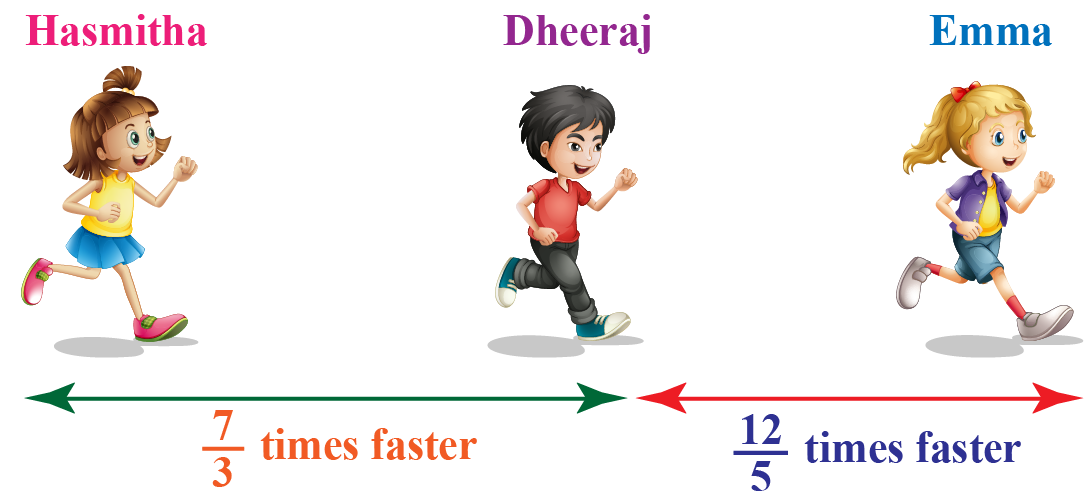Solution:

Speed of Dheeraj = \begin{align}\frac{7}{3}\end{align} times faster than Hasmitha

Speed of Emma = \begin{align}\frac{12}{5}\end{align} times faster than Dheeraj

\begin{align} \frac{12}{5}\times \frac{7}{3} = \frac{12\times 7}{5\times 3} \end{align}

(12 can be divided by 3)

We multiply the numerators and denominators.

\begin{align} \frac{4 \times 7}{5 \times 1} = \frac{28}{5} \end{align}

 $$\therefore$$ Emma runs \begin{align}\frac{28}{5}\end{align} times faster than Hasmitha.

Have a doubt that you want to clear? Get it clarified with simple solutions on Multiplication of Fractions from our Math Experts at Cuemath’s LIVE, Personalised and Interactive Online Classes.

Make your kid a Math Expert, Book a FREE trial class today!

## Practice Questions on Multiplying Fractions

Here are a few activities for you to practice.

IMO (International Maths Olympiad) is a competitive exam in Mathematics conducted annually for school students. It encourages children to develop their math solving skills from a competition perspective.

## 1. How do you multiply fractions?

To multiply any two fractions, we can follow the steps below.

• Multiply the numerators
• Multiply the denominators
• Simplify to the lowest form

## 2. How do you multiply mixed fractions?

• Change each number to an improper fraction
• Simplify if possible
• Multiply the numerators and then the denominators
• Ensure the answer is in the lowest form

## 3. How do you multiply a fraction with a whole number?

For example, $$\frac{2}{7}\times 3$$

NOTE: Multiplication of Fractions is a repeat addition of fractions

Start by rewriting the whole number (3 in this example) as a fraction, $$\frac{3}{1}$$ (You can do this because any number divided by one is always equal to itself)
Now, because you are multiplying a fraction by a fraction, you can apply the steps that you have learnt to multiply fractions.
\begin{align} \frac{2}{7}\times \frac{3}{1} = \frac{2\times 3}{7\times 1} = \frac{6}{7} \end{align}

More Important Topics
Numbers
Algebra
Geometry
Measurement
Money
Data
Trigonometry
Calculus